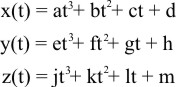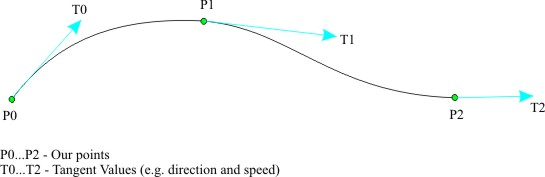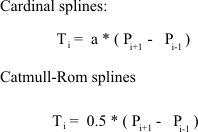Thursday February 2, 2023
 home | about | contact | DonationsThe Maths of 3D You can't have 3D without a little maths...

Cubic Splines Tutorial

by bkenwright@xbdev.net

First things first, let me say...sometimes some people call the Cubic Spline the Hermite spline....but its upto you...I prefer to call it the Cubic :)  Lets take a looksy at the 3 equations...well its just one equation...which we use for x,y and z.  Remember if you work just in the 2D...you can throw away the Z.Well t is the value we'll use to get from one point to the next...and is usually defined as 0 <= t <=1, make sure you remember that.  As when t is zero...where at the start point...and when t is one, where at our end point.  So we increment t using a small step value to get the various points in-between our two existing points.  These mini inner points will produce that curvyness.Lets take x(t) equation as an example....once we solve this, the other two are solved as well, as they are the same more or less...just different letters.  Lets solve it using basic maths, then work towards what a matrix would look like then onto a simple code demo.It looks like a lot....but it you usually do it once.  As I got out the old notepad and proved it once using a pencil and paper....usually sticks in my head better then once I've done it.

Putting it into a small C algorithm is really quite simple, as I've shown here:

 pseudo c code: code.c float x;   void Compute_x_Spline( int x0,  int x1,                                  float dx0, float dx1 ) {         int step;       float t, incr;         float a = 2*x0 - 2*x1 + dx0 + dx1;       float b = -3*x0 + 3*x1 - 2*dx0 - dx1;       float c = dx0;       float d = x0;         step = 0;       incr = 0.05f;       t = 0;       while( t<=1 )       {             x[step] = a*t*t*t + b*t*t + c*t + d;             step += 1;             t += incr;       }// End while loop   }// End Compute_x_Spline(..)         // Move to the first point on our new line xmoveto( x, y ); // Draw our new line with the curve for( int i=1; i<20; i++ )       xlineto( x[i], y[i] );

Using what we have up to know....I created a basic Win32 app using DirectX as the render surface.  Its allows us to use the right and left mouse button to control the tangent vectors for two points.  Its a really simple piece of code and would be a lot simpler if it wasn't for the win32 and DirectX surface code.

 Download Visual Studio Project Code Try holding the left or right...or even both the mouse buttons down and moving around the screen.  I've only applied the simple example to the x and y, but you can easily apply it to the 3rd dimension..!  Yup I'm talking z here...spooky z..hehehA definite must is to look at the example reference at the bottom, which gives some ideas on calculating values for our tangents automatically that look good.  Here are a few examples:-------------------------------------------------------

References:

http://www.cubic.org/~submissive/sourcerer/hermite.htm - its a nice web site, and explains more or less the same details I've gone over above.  Always best to read a few different tutorials first so you get a real feel for splines.

 Visitor: 9534626 { 229.27.38.75 } Copyright (c) 2002-2020 xbdev.net - All rights reserved. Designated articles, tutorials and software are the property of their respective owners.